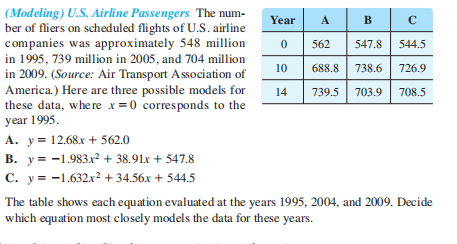### Still have math questions?

Algebra
Question(Modeling) U.S. Airline Passengers The num- ber of fliers on scheduled flights of U.S. airline companies was approximately 548million in 1995, 739million in 2005, and 704million in 2009. (Source: Air Transport A ssociation of A merica.) Here are three possible models for these data, where $$x = 0$$ corresponds to the year $$1995$$ . A. $$y = 12.68 x + 562.0$$

B. $$y = - 1.983 x ^ { 2 } + 38.91 x + 547.8$$

C. $$y = - 1.632 x ^ { 2 } + 34.56 x + 544.5$$

The table shows each equation evaluated at the years 1995,2004, and 2009 . Decide which equation most closely models the data for these years.

$$B$$